### Home > A2C > Chapter Ch4 > Lesson 4.2.4 > Problem4-127

4-127.
1. A dart hits each of these dart boards at random. What is the probability that the dart will not land in the shaded area? Homework Help ✎

1.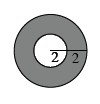2.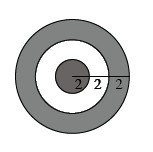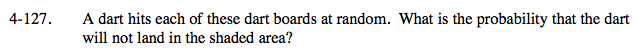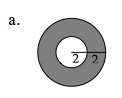P(not in shaded area) = P(white)

$P(\text{white})=\frac{\text{area of success}}{\text{total area}}=\frac{\text{area of white}}{\text{area of large circle}}$

Area = πr2

$P(\text{not in shaded area})=\frac{1}{4}$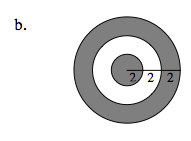See part (a).